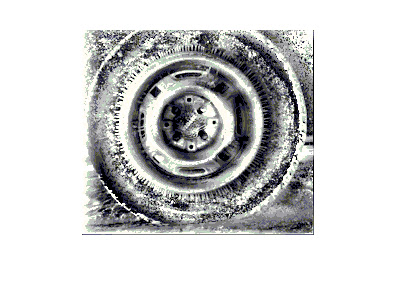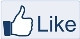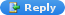# IMAGE PROCESSING

" Two roads diverged in a wood, and I,
I took the one less traveled by,
And that has made all the difference "-Robert Frost

### MATLAB CODE:Local Histogram equalization

For every pixel, based on the neighbor hood value the histogram equalization is done. Here I used 3 by 3 window matrix for explanation. By changing the window matrix size, the histogram equalization can be enhanced. By changing the values of M and N the window size can be changed in the code given below.

Steps to be performed:

MATLAB CODE:

figure,imshow(A);
Img=A;

%WINDOW SIZE
M=10;
N=20;

mid_val=round((M*N)/2);

%FIND THE NUMBER OF ROWS AND COLUMNS TO BE PADDED WITH ZERO
in=0;
for i=1:M
for j=1:N
in=in+1;
if(in==mid_val)
break;
end
end
end
%PADDING THE IMAGE WITH ZERO ON ALL SIDES

cdf=zeros(256,1);
inc=1;
for x=1:M
for y=1:N
%FIND THE MIDDLE ELEMENT IN THE WINDOW
if(inc==mid_val)
ele=B(i+x-1,j+y-1)+1;
end
pos=B(i+x-1,j+y-1)+1;
cdf(pos)=cdf(pos)+1;
inc=inc+1;
end
end

%COMPUTE THE CDF FOR THE VALUES IN THE WINDOW
for l=2:256
cdf(l)=cdf(l)+cdf(l-1);
end
Img(i,j)=round(cdf(ele)/(M*N)*255);
end
end
figure,imshow(Img);
figure,
subplot(2,1,1);title('Before Local Histogram Equalization'); imhist(A);
subplot(2,1,2);title('After Local Histogram Equalization'); imhist(Img);After Local Histogram Equalization

Histogram equalization of an Image:
http://angeljohnsy.blogspot.com/2011/04/matlab-code-histogram-equalization.htmlLike "IMAGE PROCESSING" pageAnonymous said...How can this code be modified to perhaps have a window of 7x7Anonymous said...How can this code be modified to have another "window" other than 3x3?

Aaron Angel said...@Anonymous
I have modified the code so that you can change the window size of your convenience.

rushabh said...@Aaron Angel

if i have an image of size 112X92 and i want to apply histogram based image processing what appropriate size of window should i give??

rushabh said...if i have an image of size 112X92 an i want to apply histogram based image processing
what appropriate window size should i give?

Aaron Angel said...@rushabh
Use a large window with mxn values in odd number.

kumail abbas said...SALAM...can anyone plz help me on MATLAB Code: Global Histogram Equalization on this page..i too need it..plz help me as early as u can..lotttt of thanxxx

Aaron Angel said...@kumail abbas

http://angeljohnsy.blogspot.com/2011/04/matlab-code-histogram-equalization.html

shubham matlab said...how can this code be extended to oriented local histogram equalisation
sir,
i m providing you some data
OLHE is similar to local histogram
equalization (LHE), but it captures the orientation of edges
while LHE does not. We begin with a brief review on LHE. For
each pixel on an image, we perform the histogram equalization
on the local w -by- h window centering on this pixel using
f ( x ) = (round(cd f ( x )) − min(cd f))*(L-1)/(w · h − cd f)
min

where x is the pixel intensity value, cd f ( x ) is the cumulative
distribution function of the histogram of the pixel intensities in
the w -by- h window, cd f
min is the minimum intensity in this
window, andL is the desired number of output gray levels.
Typically a square window is used, and we definek ≡ w = h .
We call the center of the k -by- k window the anchor . For LHE,
the anchor point is the pixel to be processed itself. For thewhole image, each pixel repeats the above operation and uses
f ( x ) to get its new intensity value. Fig. 1 illustrates LHE.
We define the generalized LHE operator as
L
ξ,η
k
( I W ∗ H
) = I

W ∗ H
(2)
where ξ,η is the relative position of the anchor point to
the pixel to be processed, I W ∗ H
is the input image whose
dimension is W -by- H ,andI

W ∗ H
is the histogram-equalized
image with the same dimension. The typical LHE which uses
the k -by- k local window can be denoted as L
0 , 0
k
, since the
anchor point is exactly the pixel to be processed itself.
If the pixel to be processed is brighter than all the neigh-boring pixels around it, it will have a large intensity value
after the local histogram equalization, and vice versa. We can
make LHE ‘oriented’ by changing anchor positions. Fig. 2
shows nine LHE operators using 3-by-3 windows. The eight
operators with { ξ,η } other than{ 0 , 0 } are ‘oriented’, and they
are dubbed as the Oriented Local Histogram Equalization
operators (OLHE operators) in this paper. The following
gives the formal definition of the OLHE operators:
O

k
≡ L
(
( k − 1 )
2
,
− ( k − 1 )
2
)
k
, O

k
≡ L
( 0 ,
− ( k − 1 )
2
)
k
,
O

k
≡ L
(
− ( k − 1 )
2
,
− ( k − 1 )
2
)
k
, O
−→
k
≡ L
(
( k − 1 )
2
, 0 )
k
,
O
←−
k
≡ L
(
− ( k − 1 )
2
, 0 )
k
, O

k
≡ L
(
( k − 1 )
2
,
( k − 1 )
2
)
k
,
O

k
≡ L
( 0 ,
( k − 1 )
2
)
k
, O

k
≡ L
(
− ( k − 1 )
2
,
( k − 1 )
2
)
k
(3)
where k is an odd number. Note that according to our
definition, there will always be exactly eight OLHE opera-tors no matter what the value of k is. Given an image I ,
OLHE produces 8 images, which areO

k
( I ) , O

k
( I ) , O

k
( I ) ,
O
−→
k
( I ) , O

k
( I ) , O
←−
k
( I ) O

k
( I ) and O

k
( I ) . The 8 images
are referred to as the OLHE images.

Memoona Umar said...hey can any one please help me with code for enhancement of an image of a finger because I have to extract the fingerprint from it but cant get past binzrization cz the change intensity of the pattern in image is very low so it just gives me a black screen please help.

Victor said...Great job, but i have a question.

Why on i and j you used i= 1:size(B,1)-((PadM*2)+1) and j=1:size(B,2)-((PadN*2)+1) instead of use i= 1:size(B,1) and j=1:size(B,2), for the lest elements from the right can participate on the calculation of the probability of density?

Unknown said...Nice man. really good code.

Unknown said...How should one decide window size LocalHistogram Equalisation given a different input size image?